EN | ES | DE | NL | RU

## Gases cheat Sheet

Gases Cheat Sheet

Gases

Gas is one of the phases of matter. Distances between atoms or molecules in gas phase are larger than solids and liquids.

Volume (V)

Volume of gas is equal to volume of container. They have no specific volumes. Under standard temperature and pressure 1 mole gas has 22,4 liters volume. Units of volume we use here is liter.

Temperature (T)

In calculations of gases absolute temperature (K) is used. In -273, diffusion of gases is zero and in nature Kelvin is the unit of temperature and relation between K and 0C is;

Pressure:

Pressure is the force acting perpendicularly on unit surface. Unit of pressure is mm Hg, cm Hg or atm. In general, (atmospheric pressure) atm is used.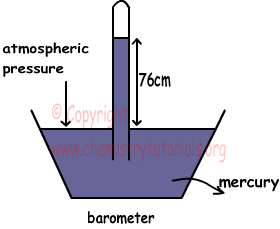Let P0 is the atmospheric pressure then;
P0=h cm Hg

P0=76 cm Hg at sea level.

h depends on;

density of the liquid put into container

h does not depend on cross section area of tube.

Manometers

Pressure of gas in a closed container is equal in everywhere. Manometers are used for measure pressure of gas in closed container.There are two types of manometers, closed end manometers and opened end manometers.

Kinetic theory is a model that deals with motion of gas atoms/molecules in a closed container.

• Gas molecules or atoms do random motions in container.
• During this random motion, they collide to each other and surface of container.
• They diffuse homogeneously in the container. If we put two different gases into same container, they produce homogeneous mixture.
• Spaces between molecules and atoms  in gases are larger than spaces between particles  in solids and liquids.
• At an instant time speeds of gas atoms/molecules are not equal. They are inversely proportional to  square root of molecular mass and directly proportional to square root of absolute temperature.
• At same temperature, average kinetic energies of all atoms/molecules are equal.
• Collisions of gas atoms/molecules to each other and surface of container are elastic, thus no energy is lost.
• Attraction between gas atoms/molecules are weak.

Diffusion:

Mixing molecules of one gas with molecules of another gas is called diffusion. Smell of a perfume or meal in a room are some common examples of diffusion of gases. Gases have different diffusion rates at different temperatures. Following formula shows ratio of diffusion rates of two gases at same temperature.

Diffusion rate (r) is directly proportional to average molecular velocity.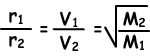Where; r1 and r2 are diffusion rates of gas 1 and gas 2, V1 and V2 are average molecular velocities of gases and M1 and M2 are molecular masses of gases.

Effusion: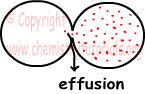As you can see from the picture given above, motion of the gases from one container to another by passing through small hole is called effusion (as given in the picture, in general second container is empty, or vacuum).Diffusion takes place under constant pressure on the contrary effusion takes place under pressure difference between containers.

Gas Laws

1. Boyle's Law:(Pressure-volume relation)

We can define Boyle's Law;

"Under constant temperature and number of particles, pressure and volume of gases are inversely proportional to each other."

V is inversely proportional to P or

P.V=constant

Moreover;

P1.V1=P2.V2=P3.V3=.. (for same gas under constant temperature and number of particles.)

2. Charles' Law:(Volume-temperature relation)

Under constant number of particles and pressure, volume of gas is directly proportional to absolute temperature. This statement is called "Charles' law".

V/T=constant (number of particles "n" and pressure constant "P")

3. Gay Lussac's / Amonton's Law:(Pressure-temperature relation)

When we increase temperature of gas, placed in a container having constant volume, speed of gas molecules increase. Increasing in the speed of molecules increase collision number to surfaces this is pressure. In other words, increasing temperature of the gas under constant volume and number of particles, increase the pressure of gas.

Thus; P1/T1=P2/T2

4. Avogadro's Law: (Volume-Number of particles relation)

Gases, having equal pressure and temperature, have equal number of particles in equal amount of volumes. In other words, volume and number of particles of gases are directly proportional to each other. We said in previous topics that 1mol gas is 22,4 liter under standard pressure and temperature and 1mol gas contains 6,02x1023 molecules/atoms.

V/n=constant or;

V1/n1=V2/n2 (P and T are constant)

5. Dalton's Law:(Pressure-number of particles relation)

Increasing number of particles in a closed container, pressure of gas increases. In other words, pressure of gases is directly proportional to moles of it under constant volume and temperature.

P/n=constant or;

P1/n1=P2/n2

n ideal gas; molecules have no volume and there are no interaction between them. In real there is no such a gas, it is just an assumption. All real gases has small volumes and there are interactions between them.

P.V=n.R.T

Where; P pressure, V volume, n number of particles, R gas constant 0,08206 L atm / K mol or 22,4/273 L atm / K mol, and T temperature

Density of gases is too small with respect to solid and gas phases. We can find density with following formula;

d(gas)=m(gas)/V(gas)

if we substitute it into the ideal gas law;

P.V=n.R.T  where n=mass/molar mass

P.V=(m/Mm).R.T

P.Mm=(m/V).R.T

P.Mm=d.R.T

d=(P.Mm)/(R.T)

If gases do not react with each other, they produce homogeneous mixture.

Partial Pressure:

Partial pressure of one of the gases in mixture placed in a closed container is equal to pressure of same gas only in same container at same temperature.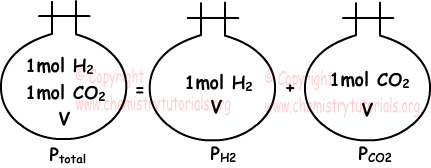Dalton's Law of Partial Pressure:

"Sum of partial pressures of the gases in the container gives us total pressure of mixture." This statement is called Dalton's law of partial pressure. Picture given above also examples of this law.

Ptotal =PH2+PCO2

Mixtures of gases ca be found with following equation;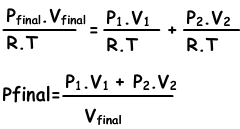Gases Exams and  Problem Solutions

The Original Author: